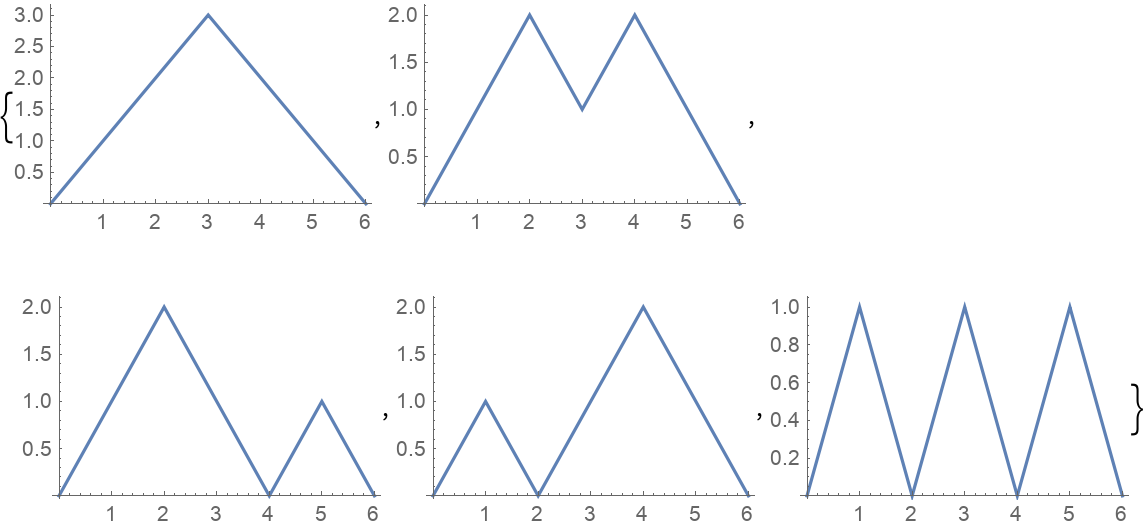#Function Repository Resource:

# DyckWords

Give all possible ways to form proper brackets

Contributed by: Wolfram Staff
 ResourceFunction["DyckWords"][n] gives all possible ways to form proper sets of n pairs of brackets.

## Details and Options

Three conditions define a proper set of brackets: (1) [ ] is a proper set; (2) two proper sets can be concatenated; and (3) a proper set can be placed anywhere inside another proper set.

## Examples

### Basic Examples

Here is the simplest nontrivial example with two pairs of brackets:

 In:=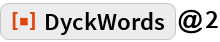Out=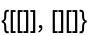Here are the ways to nest three pairs of brackets:

 In:=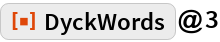Out=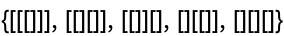There are five ways to do that:

 In:=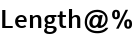Out=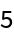Dyck words are counted by the Catalan numbers:

 In:=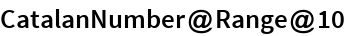Out=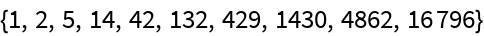### Scope

Define the helper function:

 In:=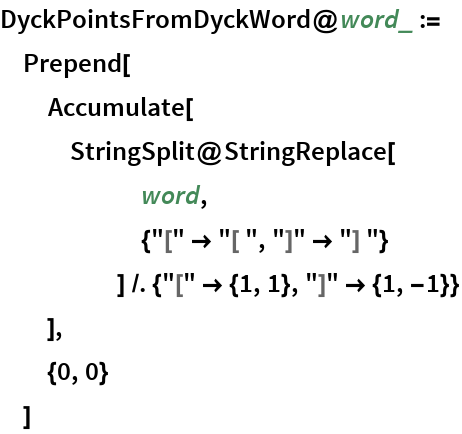A Dyck path of length 2n units starts at the origin, goes NE or SE one unit and finishes on the x axis:

 In:=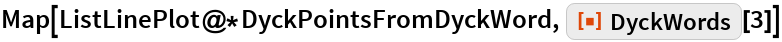Out=